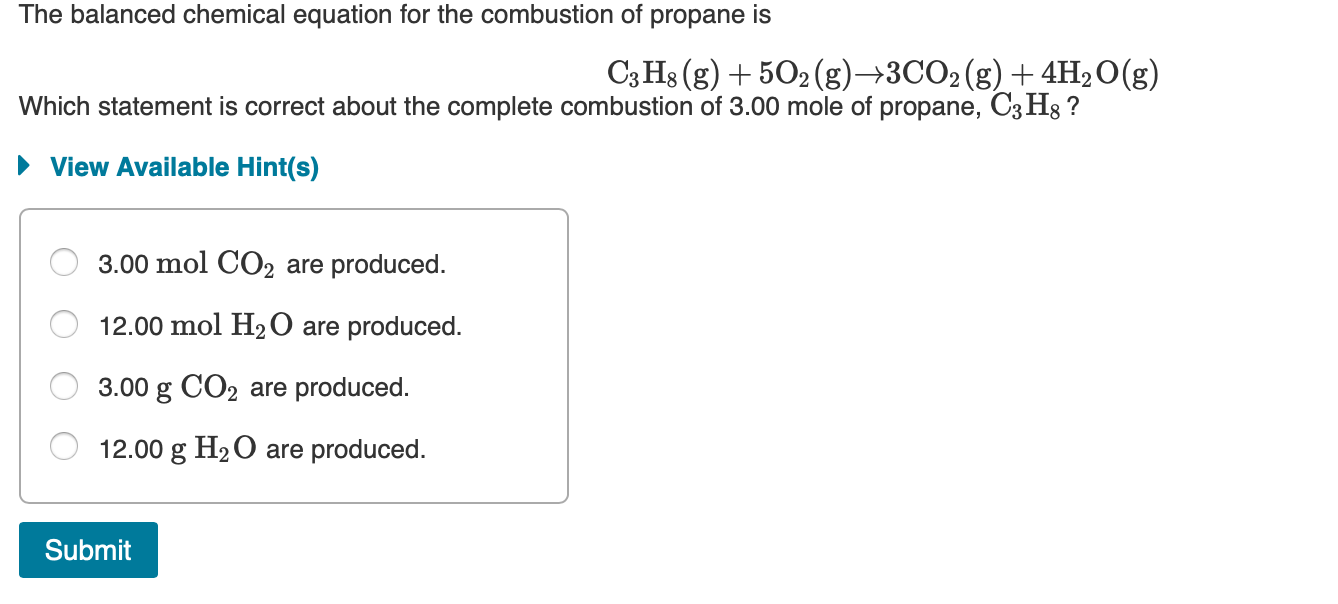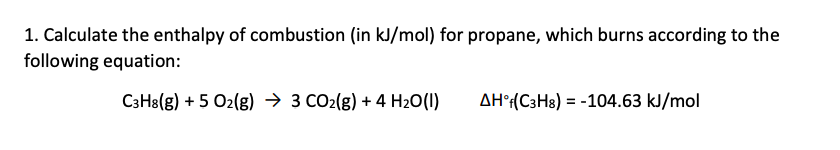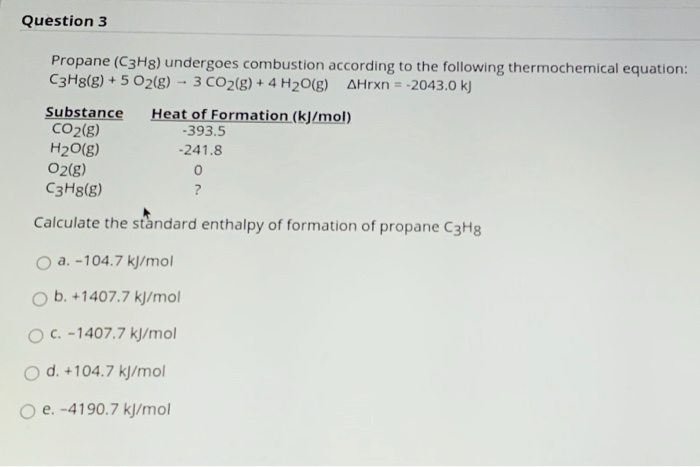Question

# calculate the enthalpy of the combustion of propane for your barbeque C3H8 + 5O2 -> 3CO2 + 4H2O propane -105 kJ...

calculate the enthalpy of the combustion of propane for your barbeque

C3H8 + 5O2 -> 3CO2 + 4H2O

propane -105 kJ/mol
CO2 -394 kJ/mol
Water -286 kJ/mol
Ooxygen. 0

Heat of formation of C3H8 , CO2 , H2O, O2 are -104 kj/mol , -393.5 kJ/ mol , -285 KJ/ mol , zero

Heat of combustion = Heat of product - Heat of reactant

= 3* -393.5 + 4 * -285 - [ -104 + 0 ] KJ

= -1180.5 + -1140 + 104 KJ

= -2216.5 KJ

#### Earn Coins

Coins can be redeemed for fabulous gifts.

Similar Homework Help Questions
• ### The combustion of propane, C3H8, occurs via the reaction C3H8(g)+5O2(g)→3CO2(g)+4H2O(g) with heat of formation values given...

The combustion of propane, C3H8, occurs via the reaction C3H8(g)+5O2(g)→3CO2(g)+4H2O(g) with heat of formation values given by the following table: Substance ΔH∘f (kJ/mol) C3H8 (g)=  -104.7 CO2(g)= −393.5 H2O(g)= −241.8 Calculate the enthalpy for the combustion of 1 mole of propane.

• ### The combustion of propane (C3H8) produces CO2 and H2O: C3H8 (g) + 5O2 (g) → 3CO2...

The combustion of propane (C3H8) produces CO2 and H2O: C3H8 (g) + 5O2 (g) → 3CO2 (g) + 4H2O (g) The reaction of 7.5 mol of O2 with 1.4 mol of C3H8 will produce ________ mol of CO2. Group of answer choices.

• ### Consider the combustion of propane: C3H8 (g) + 5O2 (g) → 3CO2 (g) + 4H2O(l) ΔH...

Consider the combustion of propane: C3H8 (g) + 5O2 (g) → 3CO2 (g) + 4H2O(l) ΔH = –2221 kJ Assume that all of the heat comes from the combustion of propane. Calculate ΔH in which 5.00 g of propane is burned in excess oxygen at constant pressure.

• ### The balanced chemical equation for the combustion of propane is C3H8(g) + 5O2(g) +3CO2(g) + 4H2O(g)...The balanced chemical equation for the combustion of propane is C3H8(g) + 5O2(g) +3CO2(g) + 4H2O(g) Which statement is correct about the complete combustion of 3.00 mole of propane, C3Hg ? ► View Available Hint(s) O 3.00 mol CO2 are produced. O 12.00 mol H2O are produced. O 3.00 g CO2 are produced. O 12.00 g H2O are produced. Submit

• ### ﻿Propane (C3H8)burns according to the following balanced equation: C3H8(g)+5O2(g)→3CO2(g)+4H2O(g) Calculate ΔH∘rxnΔ for this reaction using standard...

﻿Propane (C3H8)burns according to the following balanced equation: C3H8(g)+5O2(g)→3CO2(g)+4H2O(g) Calculate ΔH∘rxnΔ for this reaction using standard enthalpies of formation. (The standard enthalpy of formation of gaseous propane is -103.9 kJ/molkJ/mol.) Express the enthalpy in kilojoules to four significant figures.

• ### The propane fuel (C3H8) used in gas barbeques burns according to this thermochemical equation. C3H8(g)+5O2(g)→3CO2(g)+4H2O(g)ΔH∘rxn=−2044kJ If...

The propane fuel (C3H8) used in gas barbeques burns according to this thermochemical equation. C3H8(g)+5O2(g)→3CO2(g)+4H2O(g)ΔH∘rxn=−2044kJ If a pork roast must absorb 1.7×103 kJ to fully cook, and if only 15 % of the heat produced by the barbeque is actually absorbed by the roast, what mass of CO2 is emitted into the atmosphere during the grilling of the pork roast?

• ### Calculate the standard enthalpy of reaction for the combustion of propane. NOTE: This equation is not...

Calculate the standard enthalpy of reaction for the combustion of propane. NOTE: This equation is not balanced. Round to the nearest whole number. C3H8(g) + O2 --> CO2(g) + H2O(l) kJ/mol Compound Hf (kJ/mole) C3H8(g) -105 CO2(g) -394 H2O(l) -284

• ### 1. Calculate the enthalpy of combustion (in kJ/mol) for propane, which burns according to the following...1. Calculate the enthalpy of combustion (in kJ/mol) for propane, which burns according to the following equation: C3H8(g) + 5 O2(g) + 3 CO2(g) + 4H2O(1) AH® (C3H8) = -104.63 kJ/mol

• ### Question 3 Propane (C3H8) undergoes combustion according to the following thermochemical equation: C3H8(g) + 5 O2(g)...Question 3 Propane (C3H8) undergoes combustion according to the following thermochemical equation: C3H8(g) + 5 O2(g) -- 3 CO2(g) + 4H2O(g) Arxn = -2043.0 kJ Substance Heat of Formation (kJ/mol) CO2(g) -393.5 H2O(g) -241.8 O2(g) 0 C3H8(g) ? Calculate the standard enthalpy of formation of propane C3H8 a. -104.7 kJ/mol ob. +1407.7 kJ/mol C. -1407.7 kJ/mol O d. +104.7 kJ/mol o e. -4190.7 kJ/mol

• ### The propane fuel (C3H8) used in gas barbeques burns according to the following thermochemical equation: C3H8(g)+5O2(g)...

The propane fuel (C3H8) used in gas barbeques burns according to the following thermochemical equation: C3H8(g)+5O2(g)?3CO2(g)+4H2O(g) If a pork roast must absorb 1700kJ to fully cook, and if only 14% of the heat produced by the barbeque is actually absorbed by the roast, what mass of CO2 is emitted into the atmosphere during the grilling of the pork roast? Express your answer using two significant figures.